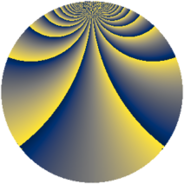# Properties

 Label 1849.4.mLevel $1849$ Weight $4$ Character orbit 1849.m Rep. character $\chi_{1849}(4,\cdot)$ Character field $\Q(\zeta_{301})$ Dimension $118944$ Sturm bound $630$

# Related objects

## Defining parameters

 Level: $$N$$ $$=$$ $$1849 = 43^{2}$$ Weight: $$k$$ $$=$$ $$4$$ Character orbit: $$[\chi]$$ $$=$$ 1849.m (of order $$301$$ and degree $$252$$) Character conductor: $$\operatorname{cond}(\chi)$$ $$=$$ $$1849$$ Character field: $$\Q(\zeta_{301})$$ Sturm bound: $$630$$

## Dimensions

The following table gives the dimensions of various subspaces of $$M_{4}(1849, [\chi])$$.

Total New Old
Modular forms 119448 119448 0
Cusp forms 118944 118944 0
Eisenstein series 504 504 0

## Trace form

 $$118944q - 249q^{2} - 255q^{3} + 1621q^{4} - 235q^{5} - 274q^{6} - 354q^{7} - 319q^{8} + 4077q^{9} + O(q^{10})$$ $$118944q - 249q^{2} - 255q^{3} + 1621q^{4} - 235q^{5} - 274q^{6} - 354q^{7} - 319q^{8} + 4077q^{9} - 197q^{10} - 351q^{11} - 291q^{12} - 369q^{13} + 1546q^{14} - 367q^{15} + 7093q^{16} - 431q^{17} + 156q^{18} - 3767q^{19} + 105q^{20} - 170q^{21} - 679q^{22} + 3063q^{23} + 679q^{24} + 11079q^{25} - 397q^{26} - 231q^{27} - 287q^{28} + 109q^{29} + 4217q^{30} - 2469q^{31} - 693q^{32} + 2336q^{33} + 325q^{34} - 4688q^{35} - 101602q^{36} - 1278q^{37} - 1165q^{38} + 1313q^{39} - 1521q^{40} - 471q^{41} + 1572q^{42} - 1919q^{43} - 5810q^{44} - 1277q^{45} + 2528q^{46} - 95q^{47} - 163q^{48} - 136858q^{49} + 24q^{50} - 1782q^{51} + 1189q^{52} + 2309q^{53} + 2489q^{54} + 1477q^{55} + 321q^{56} - 152q^{57} - 593q^{58} - 809q^{59} - 647q^{60} + 81q^{61} - 1181q^{62} + 444q^{63} + 34473q^{64} - 2721q^{65} - 55618q^{66} + 141q^{67} + 4233q^{68} - 2969q^{69} + 7440q^{70} + 2173q^{71} - 36854q^{72} + 1899q^{73} + 1134q^{74} + 27q^{75} + 6766q^{76} + 3704q^{77} + 2645q^{78} - 2910q^{79} - 11876q^{80} + 25104q^{81} - 46077q^{82} + 2612q^{83} - 3074q^{84} + 3188q^{85} - 10313q^{86} - 20998q^{87} - 10215q^{88} + 5553q^{89} - 49696q^{90} + 502q^{91} - 4874q^{92} - 4252q^{93} - 29535q^{94} + 4148q^{95} + 2808q^{96} + 37709q^{97} + 8783q^{98} + 16354q^{99} + O(q^{100})$$

## Decomposition of $$S_{4}^{\mathrm{new}}(1849, [\chi])$$ into newform subspaces

The newforms in this space have not yet been added to the LMFDB.